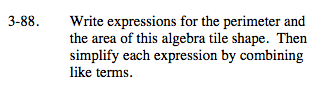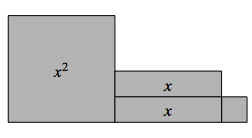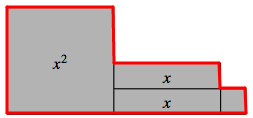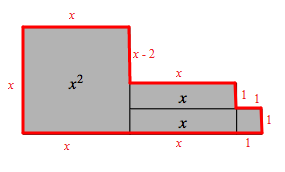### Home > MC2 > Chapter 3 > Lesson 3.2.3 > Problem3-88

3-88.The perimeter is the distance around a shape.

Label the values of all the sides.

Add these values together to find the expression for the perimeter.
x + x + (x − 2) + x + 1 + 1 + 1 + 1 + x + x
Simplify this expression by combining like terms.

The area is the space bound within the perimeter.

Add the areas of all 4 tiles within the perimeter.
x2 + x + x + 1
Now simplify this expression.P = 6x + 2